CATEGORIES:

# Work fulfillment order

1. Motor with phase rotor start.

1.1. To construct the motor scheme with phase rotor (see fig. 5.4, а).

1.2. To implement the motor startup at the maximal resistance of starting rheostat (rotor winding is short-circuited). To measure the current by ammeter with scale limit (7÷8)I. To measure acceleration time.

1.3. To implement the startup of the motor using starting rheostat and to measure starting current using two different resistances.

To compare acceleration time of induction motor from the points 1.2 and 1.3.

2. Motor with short-circuited rotor startup.

2.1. To construct the scheme (fig. 5.4, b).

2.2. To measure starting current in case of direct startup. For this is established voltage U, when current of the stator is rated I1rated (rotor is motionless). Starting current is calculated by the formula: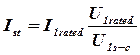Rated voltage and current are determined by the motor passport.

2. 3. To make induction motor direct startup. To set up voltage 220 V by autotransformer according to scheme, which shown in fig. 5.4, b. Switch-off motor and wait till rotor stops. Put ammeter with scale (7÷8)Iinto the circuit and measure Inet, and compare it with current Inet, which was obtained at the item 2.2.

2.4. Start with autotransformer. According to scheme (which is shown in fig. 5.4, b) set up the voltage (U1=127 V) and determine transformation ratio by the formula:To connect a motor and carry out start at U2 = 127 V, to fix the current of motor Imotor and current, consumed from the network Inet, to check up correlation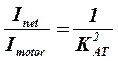.

To make the conclusion about the starting torque value change during startup of the motor through an autotransformer in comparison with the direct start.

2.5. Start with the help of delta-star switching stator winding of induction motor. Starting scheme is presented in figure 5.4, c.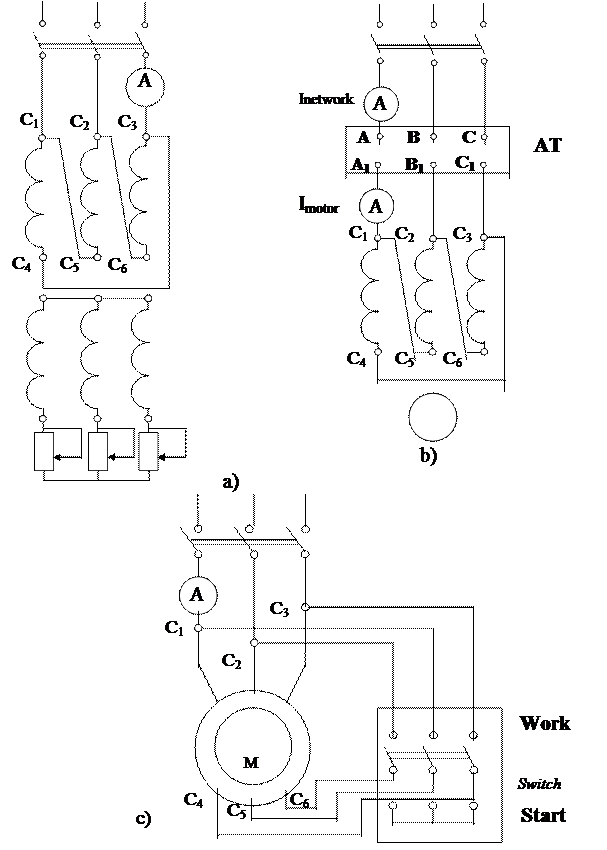Figure 5.4 - Induction motors start schemes

If switch 2 is in position "Start", the stator winding is star-connected, if in position "Work", the stator winding is delta-conneсted.

Startup is performed as following. We put a switch 2 in position "start”, then we switch-on autotransformer and fix the starting current Ist. When a motor reaches the established speed, it is necessary to move quickly a switch 2 in position "Work". Startup is finished.

After it you must switch-off autotransformer and wait, when motor stops. To switch-on autotransformer and to fix starting current at delta connection of stator windings. Make sure that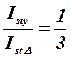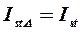Make the conclusion of starting torque value, by measuring of the phase voltages in the star or delta connection of the stator winding

Basic results summary

 Motor Current According to the passport Irated Direct start Inet (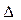) At rm1 Inet At rm2 Inet Start with autotransformer Inet At star connection Inet Phase rotor Short-circuited rotor

Control questions

1. Why is starting current higher than nominal at the startup?

2. Why is current decreased during the frequency of rotation of the motor increasing?

3. Why is starting current decreased in the induction motor having the phase rotor, when starting resistance is introduced?

4. How is the value of starting torque of motor are changed, if as the starting resistance active or inductive resistance is used?

5. How is starting torque changed in different types of induction motor startups?

6. How is acceleration of motor time is changed in depending on the start method?

Report must contain:

1. Experiments schemes and measurements and calculations results.

2. Summary of basic results.